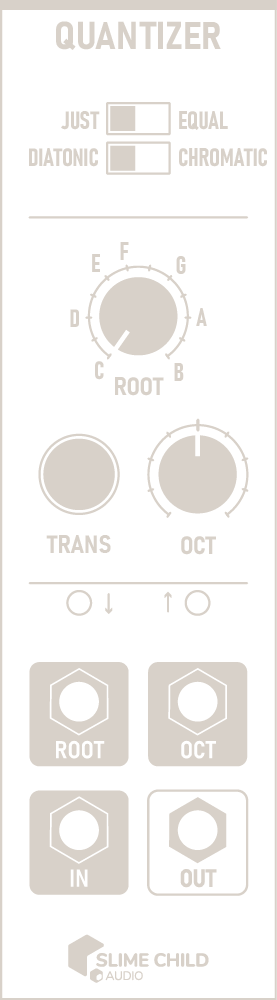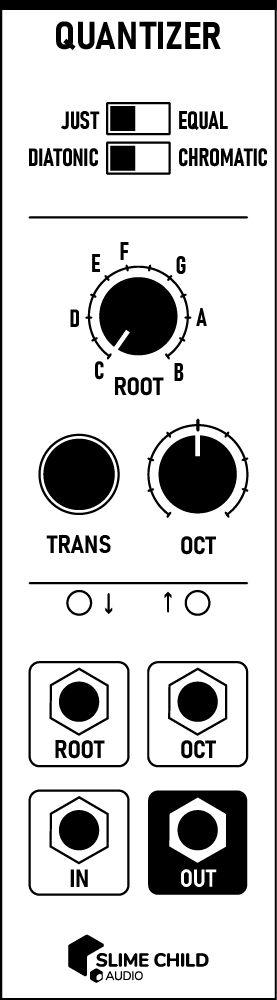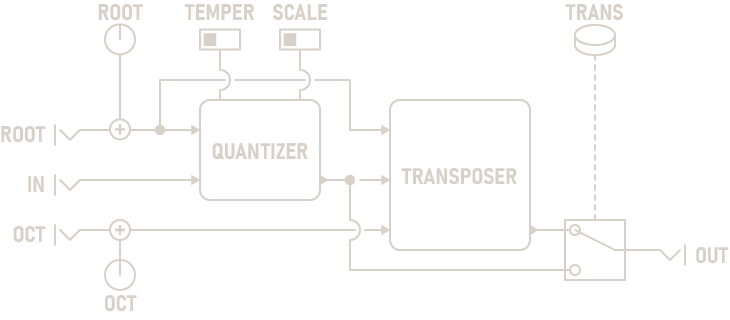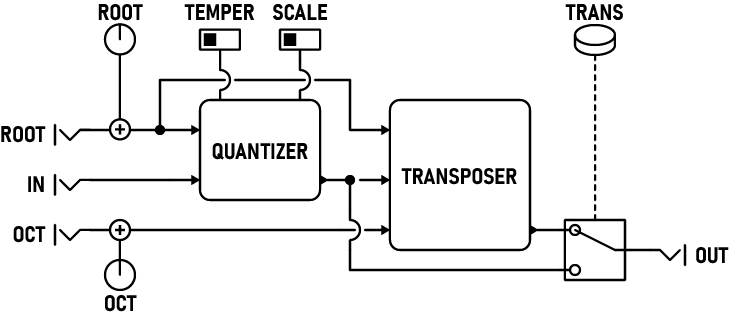## Description

The Quantizer takes in a continuous pitch and adjusts it to match a musical scale. Using a quantizer, you can ensure that an oscillator will harmonize with other tonal instruments. This module can quantize using more than three dozen different scales, depending on your chosen scale degree, temperament, and root.

While the ROOT knob specifies the root of a major scale, this module can also be used to quantize to minor scales. To use a minor scale, set the root to the scale's relative major scale, e.g. to use A minor, set the root to C.

In EQUAL Temperament mode, input is quantized to either a chromatic (12-step) scale or one of twelve different diatonic (8-step, major) scales. These scales use the standard equal temperament tuning used by most modern instruments, where all semitones are exactly 100 cents apart.

In JUST Intonation mode, the quantizer uses an alternate tuning based on integer ratios instead: C=11, G=32, F=43, etc. Because this temperament uses whole number fractions, any subharmonics generated by the Sub-Oscillator module will sound more in-tune.

Note: A 5-limit asymmetric chromatic extension of Ptolemy's intense diatonic scale was used to construct this scale. Notes differ from equal temperament by up to 18 cents depending on the root note.

## Parameters

• ### TEMPERAMENT Switch

Switches between just intonation and equal temperament.

• ### SCALE DEGREE Switch

Set the scale degree to quantize to. When set to DIATONIC, an eight-note major scale is used; when set to CHROMATIC, a twelve-tone chromatic scale is used.

• ### ROOT Knob

Set the root note of the quantization scale. This ordinarily represents the root of a major scale. To use a minor scale, set the root to the scale's relative major scale, e.g. to use A minor, set the root to C.

Note: This knob has no effect when TEMPERAMENT is set to EQUAL and SCALE DEGREE is set to CHROMATIC.

• ### TRANS Button

If set, transposes the quantized note so that 0V corresponds to the root note set by the ROOT knob.

• ### OCT Knob

Transposes the quantized note up or down by an octave, from -4 to +4 octaves.

## Ports

• ### ROOT Input

Modifies the scale root using a 1-volt/octave pitch. Only the fractional (that is, after the decimal point) part of the voltage is used; the octave is ignored. To use a specific note and octave in conjunction, connect the ROOT and OCTAVE inputs to the same source.

V/OCT INPUT: -5V to +5V

• ### OCT Input

Adds or subtracts octaves from the output using a 1-volt/octave pitch. Only the integral (that is, before the decimal point) part of the voltage is used; the note is ignored. To use a specific note and octave in conjunction, connect the ROOT and OCTAVE inputs to the same source.

V/OCT INPUT: -5V to +5V

• ### IN Polyphonic Input

Accepts a 1-volt/octave pitch to quantize.

V/OCT INPUT: -5V to +5V, Polyphonic

• ### OUT Polyphonic Output

Outputs the quantized pitch.

V/OCT OUTPUT: -5V to +5V, Polyphonic